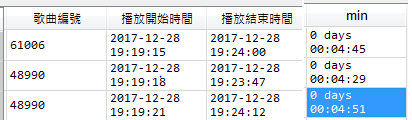#2018 iT 邦幫忙鐵人賽DAY 8
1
Data Technology

## [Day08]Pandas資料的取得與篩選！

[Day06]Pandas的兩種資料類型！
[Day07]Pandas操作資料的函數！

# 資料取得

## Series

``````seriesObj = pd.Series(range(10),index=['a', 'a', 'b', 'b', 'b','c','c','c','c','c'])
``````

``````import pandas as pd
``````

``````seriesObj['c']
``````

``````c    5
c    6
c    7
c    8
c    9
dtype: int64
``````

## Dataframe

``````df = pd.DataFrame(np.random.randn(4,2),index=['a', 'a', 'b', 'b'])
``````

``````import numpy as np
``````

``````import numpy as np
df = pd.DataFrame(np.random.randn(4,2),index=['a', 'a', 'b', 'b'])
df
````````````df.ix['b']
``````

# 資料篩選

[Day03]Python的基本運算！（上）
[Day04]Python的基本運算！（下）### 運算子運用

``````employees["Gender"] == "Male"
``````

``````fliter = (employees["Gender"] == "Male")
employees[fliter]
``````

``````mask = employees['Team'] != "Finance"
``````

``````employees["Salary"] > 148941
employees[employees["Salary"] > 148941]
``````

``````mask = employees["Start Date"] <= "1980-05-01"
employees[mask]
````````````mask1 = employees["Team"] != "Marketing"
mask2 = employees["Start Date"] < "1980-01-01"
``````

``````employees[(mask1 & mask2)]
``````

``````employees[(mask1 ｜ mask2)]
``````

### .isin()

``````mask = employees["Team"].isin(["Legal","Scales","Product"])
``````

### innull() & notnull()

`isnull()`如果值是空的就會顯示`True`

``````mask = employees["Team"].isnull()
``````

`notnull()`如果值不是空的就會顯示True

``````mask = employees["Team"].notnull()
``````

### between()

``````employees[employees["Start Date"].between("2009-11-10","2010-01-20")]
``````

``````employees[employees["Start Date"].between("2009-11-10","2010-01-20")]
``````## 總結

### 4 則留言

0

song['min']=pd.to_datetime(song['播放結束時間']) - pd.to_datetime(song['播放開始時間'])
filter = (song["min"] > "59") #秒數格式錯誤
song[filter]0

employees[(mask1 ｜ mask2)] 和 employees[(mask1 & mask2)]

employees[(mask1) ｜ (mask2)]

employees[(mask1) & (mask2)]

0

EX:新增一欄"狀態"，而本來的DataFrame有欄位"目前合約狀態"，"退租原因"
1.如果目前合約狀態 != 欠停、強停、合約終止 →那麼新的一行"狀態" 要顯示"繼續使用"
2.如果新的一行"狀態"為空白 &(且) 退租原因 != 欠拆、強拆、空白 → 那麼"狀態"要顯示 = "不使用"